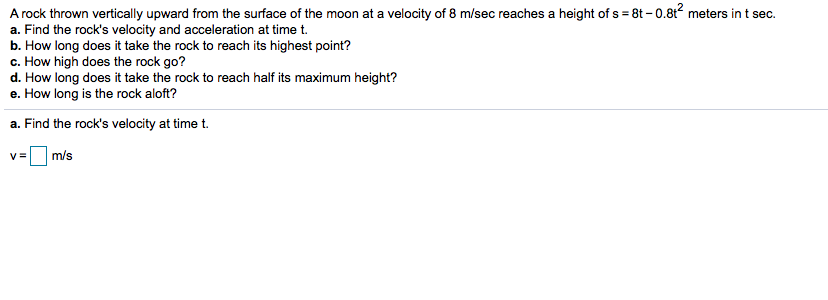# A rock thrown vertically upward from the surface of the moon at a velocity of 8 m/sec reaches a height of s 8t -0.8t2 meters in t seca. Find the rock's velocity and acceleration at time t.b. How long does it take the rock to reach its highest point?c. How high does the rock go?d. How long does it take the rock to reach half its maximum height?e. How long is the rock aloft?a. Find the rock's velocity at time t.m/sV=

Question
12 views

Can you help me step by step with this question pleasehelp_outlineImage TranscriptioncloseA rock thrown vertically upward from the surface of the moon at a velocity of 8 m/sec reaches a height of s 8t -0.8t2 meters in t sec a. Find the rock's velocity and acceleration at time t. b. How long does it take the rock to reach its highest point? c. How high does the rock go? d. How long does it take the rock to reach half its maximum height? e. How long is the rock aloft? a. Find the rock's velocity at time t. m/s V= fullscreen
check_circle

Step 1

Given information:

A rock thorwn veritcally upward from  the surface of the moon at a velocity of 8 m/sec reaches of height os s=8t-0.08t^2 in t sec.

Step 2

Calculation:

Diffeentiate the  function s w...

### Want to see the full answer?

See Solution

#### Want to see this answer and more?

Solutions are written by subject experts who are available 24/7. Questions are typically answered within 1 hour.*

See Solution
*Response times may vary by subject and question.
Tagged in

### Calculus